## How to Find the Length of Arc and the Area of Sector

### What is the Area of the Sector of a Circle?

The area of a sector in the region that a circle's sector encloses. Minor and major sectors are the two different categories of sectors. A major sector is larger than a semi-circle, whereas a minor sector is smaller than a semi-circle.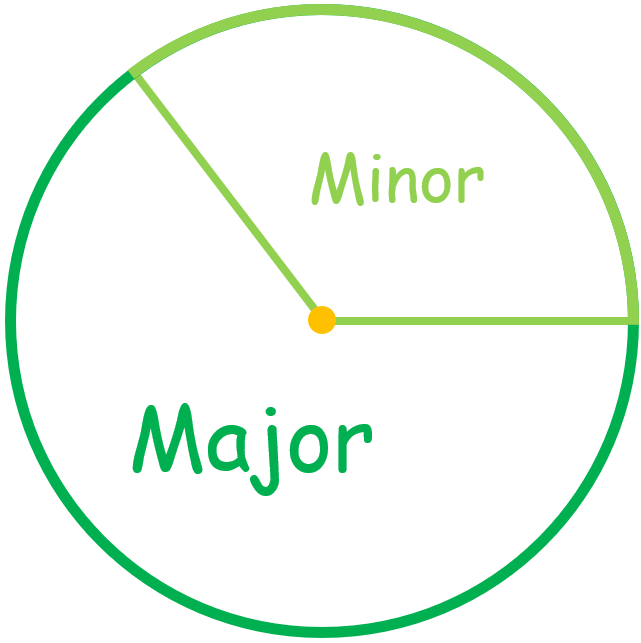### Sector Area Formula

We use the area of a sector formula to get the overall area that the sector encompasses. The following formulae can be used to determine a sector's area:

Area of a Sector of a Circle $$= \ (\frac{θ}{360}) \times πr^2$$, where $$r$$ is the circle's radius and $$θ$$ is the sector angle, in degrees, that the arc at the center subtends.

Area of a Sector of a Circle $$= \ \frac{1}{2} \times r^2θ$$, where $$r$$ is the circle's radius and $$θ$$ sector angle, expressed in radians that the arc at the circle's center subtends.

### Arc Length

Any section of a circle's circumference is considered an arc. The length of an arc is the distance between its two endpoints. Knowing a little bit about circle geometry is necessary to determine an arc length. Since the arc is a component of the circumference, you can determine the arc length if you know what percentage of $$360$$ degrees the arc's central angle is.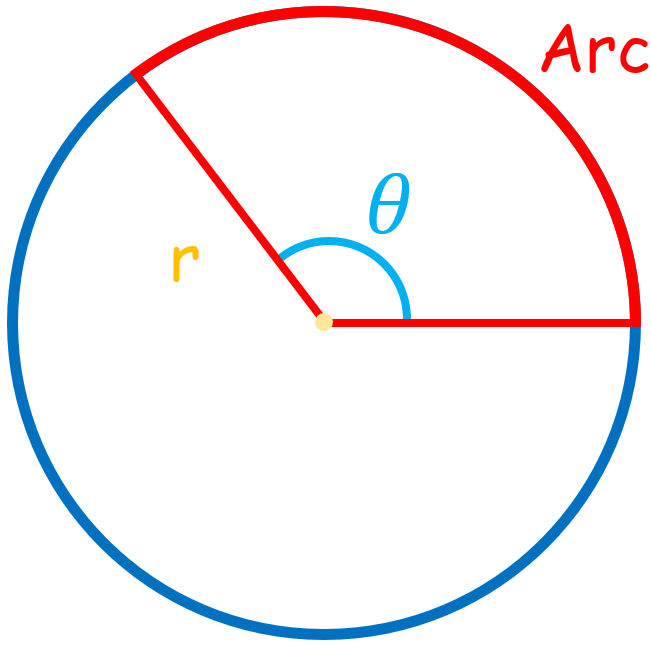### Arc Length Formula

Arc length $$= \ \frac{Central \ Angle}{360°} \times 2πr$$

### Example

Find the length of the red arc. ($$π \ = \ 3.14$$)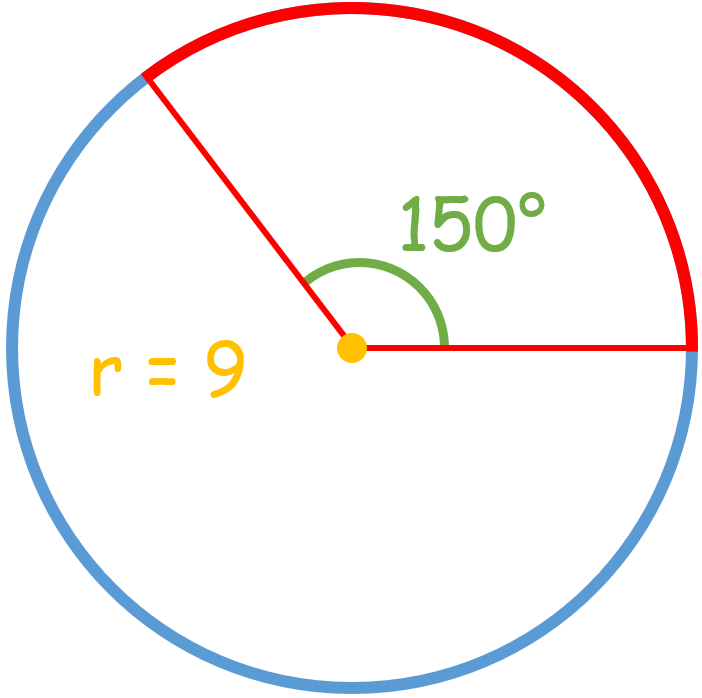Solution

Arc length $$= \ \frac{Central \ Angle}{360°} \times 2πr$$

As shown in the above picture, $$r \ = \ 9$$ and Central Angle $$= \ 150°$$, So:

Arc length $$= \ \frac{150°}{360°} \times 2π \times 9$$ $$= \ \frac{5}{12} \times 2(3.14) \times 9 \ = \ 23.55$$

Therefore, the arc length is $$23.55$$.

### Exercises for Finding the Arc Length and the Sector Area

1) Find the arc length.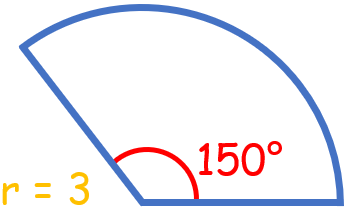2) Find the arc length.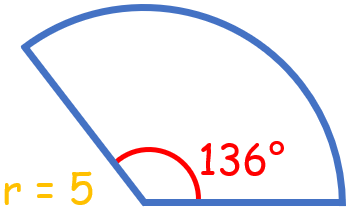3) Find the arc length.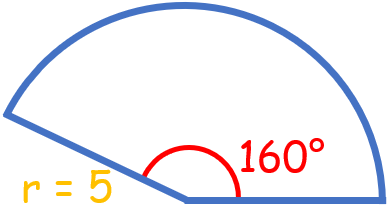4) Find the arc length.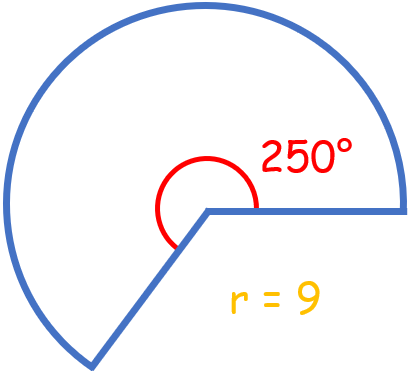5) Find the arc length.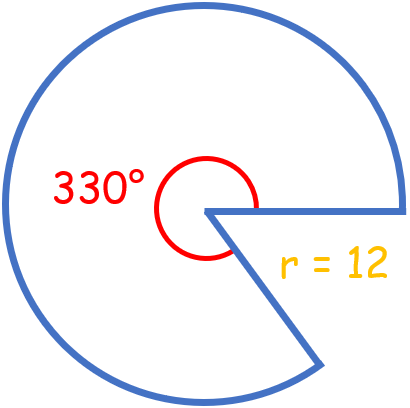6) Find the sector area.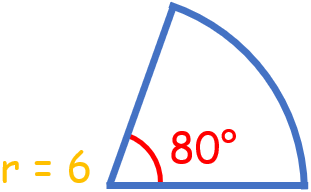7) Find the sector area.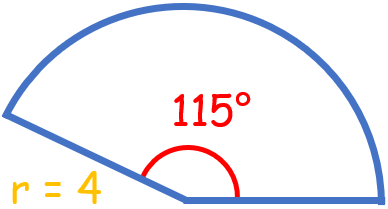8) Find the sector area.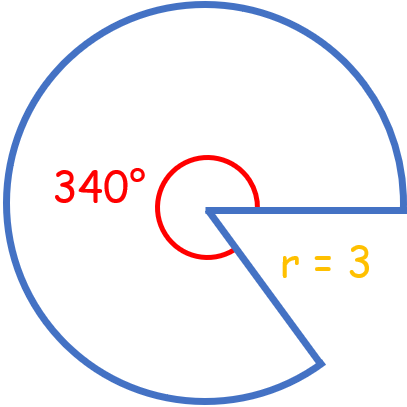9) Find the sector area.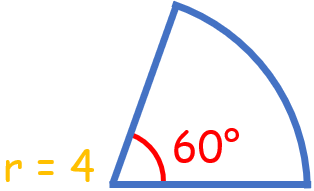10) Find the sector area.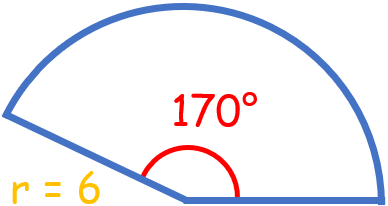1) Find the arc length.$$\color{red}{arc \ length \ = \ \frac{150}{360} \times 2π(3) \ = \ \frac{5π}{2} \ ≈ \ 7.85}$$

2) Find the arc length.$$\color{red}{arc \ length \ = \ \frac{136}{360} \times 2π(5) \ = \ \frac{34π}{9} \ ≈ \ 11.862}$$

3) Find the arc length.$$\color{red}{arc \ length \ = \ \frac{160}{360} \times 2π(5) \ = \ \frac{40π}{9} \ ≈ \ 13.955}$$

4) Find the arc length.$$\color{red}{arc \ length \ = \ \frac{250}{360} \times 2π(9) \ = \ \frac{25π}{2} \ ≈ \ 39.25}$$

5) Find the arc length.$$\color{red}{arc \ length \ = \ \frac{330}{360} \times 2π(12) \ = \ 22π \ ≈ \ 69.08}$$

6) Find the sector area.$$\color{red}{sector \ area \ = \ \frac{80}{360} \times π(6)^2 \ = \ 8π \ ≈ \ 25.12}$$

7) Find the sector area.$$\color{red}{sector \ area \ = \ \frac{115}{360} \times π(4)^2 \ ≈ \ 16.048}$$

8) Find the sector area.$$\color{red}{sector \ area \ = \ \frac{340}{360} \times π(3)^2 \ = \ \frac{17π}{2} \ ≈ \ 26.69}$$

9) Find the sector area.$$\color{red}{sector \ area \ = \ \frac{60}{360} \times π(4)^2 \ = \ \frac{8π}{3} \ ≈ \ 8.373}$$

10) Find the sector area.$$\color{red}{sector \ area \ = \ \frac{170}{360} \times π(6)^2 \ = \ 17π \ ≈ \ 53.38}$$

## Arc Length and Sector Area Practice Quiz

### GED Math for Beginners 2022

$28.99$14.99

### SAT Math Study Guide

$19.99$14.99

### GED Math Practice Workbook 2022

$27.99$13.99

### The Most Comprehensive SSAT Upper Level Math Preparation Bundle

$76.99$36.99